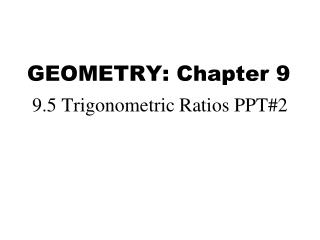DownloadDownload PresentationGEOMETRY: Chapter 9

# GEOMETRY: Chapter 9

Télécharger la présentation## GEOMETRY: Chapter 9

- - - - - - - - - - - - - - - - - - - - - - - - - - - E N D - - - - - - - - - - - - - - - - - - - - - - - - - - -
##### Presentation Transcript

1. GEOMETRY: Chapter 9 9.5 Trigonometric Ratios PPT#2

2. Sine and Cosine Ratios Image taken from: Geometry. McDougal Littell: Boston, 2007. P. 473.

3. Ex.1: Find sin A and sin B. Write each answer as a fraction and as a decimal rounded to four places. Image taken from: Geometry. McDougal Littell: Boston, 2007. P. 474.

4. Ex.1: Find sin A and sin B. Write each answer as a fraction and as a decimal rounded to four places. Answer: sin A = 8/17 ~ 0.4706 sin B= 15/17~0.8824 Image taken from: Geometry. McDougal Littell: Boston, 2007. P. 474.

5. Ex. 2: Find cos P and cos R. Write each answer as a fraction and as a decimal rounded to four places. Images taken from: Geometry. McDougal Littell: Boston, 2007. P. 474.

6. Ex. 2: Find cos P and cos R. Write each answer as a fraction and as a decimal rounded to four places. Answer: cos P =√5/3~0.7454; cos R=2/3~0.6667 Images taken from: Geometry. McDougal Littell: Boston, 2007. P. 474.

7. Ex. 3: A rope, staked 20 feet from the base of a building, goes to the roof and forms an angle of 58 degrees with the ground. To the nearest tenth of a foot, how long is the rope? Images taken from: Geometry. McDougal Littell: Boston, 2007. P. 475.

8. Ex. 3: A rope, staked 20 feet from the base of a building, goes to the roof and forms an angle of 58 degrees with the ground. To the nearest tenth of a foot, how long is the rope? Answer: 37.7 ft Images taken from: Geometry. McDougal Littell: Boston, 2007. P. 475.

9. Angles—If you look up at an object, the angle your line of sight makes with a horizontal line is called the angle of elevation. If you look down at an object, the angle your line of sight makes with a horizontal line is called the angle of depression. Images taken from: Geometry. McDougal Littell: Boston, 2007. P. 475.

10. Ex. 4: A pilot is looking at an airport from her plane. The angle of depression is 290. If the plane is at an altitude of 10,000 feet, approximately how far is it from the airport? Answer: 20,627 ft

11. Ex. 5: A dog is looking at a squirrel at the top of a tree. The distance between the two animals is 55 feet and the angle of elevation is 640. How high is the squirrel and how far is the dog from the base of the tree? Answer: 49.4ft high; 24.1 ft from the tree

12. 9.5 p. 562-563 #3-36 all